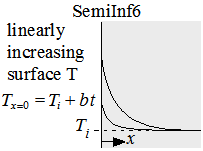Contents - Index

Linearly-increasing Surface TemperatureThe function SemiInf6(T_i, b, alpha, x, time) returns the temperature within a semi-infinite body in which the surface temperature is increasing linearly with time.  Solution is from Myers (1998).

The calling protocol is:

T = SemiInf6(T_i, b, alpha, x, time)

Inputs:
T_i = initial temperature of the material [C] or [K]
b = rate at which temperature is rising [C/s] or [K/s]
alpha = thermal diffusivity [m^2/s]
x = position relative to surface [m]
time = time relative to beginning of surface disturbance [s]

This function can be used with English units set in EES.  In this case, T_i are in [F] or [R], b is in [F/s] or [R/s], alpha is in [ft^2/hr], x is in [ft], and time is in [s].

Outputs:
T is the temperature in [C] or [K] (or [F] or [R] in English units)

Myers, G. E., Analytical Methods in Conduction Heat Transfer, 2nd Edition, AMCHT Publications, (1998).

Example:
\$UnitSystem SI Mass J K Pa
\$VarInfo T units=K
T_i=300 [K]
b=3 [K/s]
alpha=1e-4 [m^2/s]
x=0.1 [m]
time=100 [s]
T=semiinf6(T_i, b, alpha,x,time)

{Solution:
T=384 [K]}

Transient Conduction Index